tag:www.digitalocean.com,2005:/community/tags/python-string.atom DigitalOcean Community | Python String tutorials, questions and resources 2023-01-27T19:24:20.462Z DigitalOcean <![CDATA[How To Compare Strings in Python]]> tag:www.digitalocean.com,2005:/community/tutorials/python-string-comparison 2022-12-22T03:12:42.531Z Introduction

You can compare strings in Python using the equality (`==`) and comparison (`<`, `>`, `!=`, `<=`, `>=`) operators. There are no special methods to compare two strings. In this article, you’ll learn how each of the operators work when comparing strings.

Python string comparison compares the characters in both strings one by one. When different characters are found, then their Unicode code point values are compared. The character with the lower Unicode value is considered to be smaller.

## Python Equality and Comparison Operators

Declare the string variable:

``````fruit1 = 'Apple'
``````

The following table shows the results of comparing identical strings (`Apple` to `Apple`) using different operators.

Operator Code Output
Equality `print(fruit1 == 'Apple')` `True`
Not equal to `print(fruit1 != 'Apple')` `False`
Less than `print(fruit1 < 'Apple')` `False`
Greater than `print(fruit1 > 'Apple')` `False`
Less than or equal to `print(fruit1 <= 'Apple')` `True`
Greater than or equal to `print(fruit1 >= 'Apple')` `True`

Both the strings are exactly the same. In other words, they’re equal. The equality operator and the other equal to operators return `True`.

If you compare strings of different values, then you get the exact opposite output.

If you compare strings that contain the same substring, such as `Apple` and `ApplePie`, then the longer string is considered larger.

## Comparing User Input to Evaluate Equality Using Operators

This example code takes and compares input from the user. Then the program uses the results of the comparison to print additional information about the alphabetical order of the input strings. In this case, the program assumes that the smaller string comes before the larger string.

``````fruit1 = input('Enter the name of the first fruit:\n')
fruit2 = input('Enter the name of the second fruit:\n')

if fruit1 < fruit2:
print(fruit1 + " comes before " + fruit2 + " in the dictionary.")
elif fruit1 > fruit2:
print(fruit1 + " comes after " + fruit2 + " in the dictionary.")
else:
print(fruit1 + " and " + fruit2 + " are the same.")
``````

Here’s an example of the potential output when you enter different values:

``````OutputEnter the name of first fruit:
Apple
Enter the name of second fruit:
Banana
Apple comes before Banana in the dictionary.
``````

Here’s an example of the potential output when you enter identical strings:

``````OutputEnter the name of first fruit:
Orange
Enter the name of second fruit:
Orange
Orange and Orange are the same.
``````

Note: For this example to work, the user needs to enter either only upper case or only lower case for the first letter of both input strings. For example, if the user enters the strings `apple` and `Banana`, then the output will be `apple comes after Banana in the dictionary`, which is incorrect.

This discrepancy occurs because the Unicode code point values of uppercase letters are always smaller than the Unicode code point values of lowercase letters: the value of `a` is 97 and the value of `B` is 66. You can test this yourself by using the `ord()` function to print the Unicode code point value of the characters.

## Conclusion

In this article you learned how to compare strings in Python using the equality (`==`) and comparison (`<`, `>`, `!=`, `<=`, `>=`) operators. Continue your learning about Python strings.

]]>
Pankaj 2022-12-22T03:12:42.531Z
<![CDATA[How To Use Python Raw String]]> tag:www.digitalocean.com,2005:/community/tutorials/python-raw-string 2022-12-20T18:00:31.080Z Introduction

You can create a raw string in Python by prefixing a string literal with `r` or `R`. Python raw string treats the backslash character (\) as a literal character. Raw string is useful when a string needs to contain a backslash, such as for a regular expression or Windows directory path, and you don’t want it to be treated as an escape character. This article covers the basics of how Python raw strings work and provides a few common examples of how to use raw strings to include special characters in strings.

The examples in this article use the Python interactive console in the command line to demonstrate different raw string scenarios.

## Including a Newline Character in a String Using Raw String

This example uses a string with a value: `Hi\nHello`. If you try to assign this value to a normal string, then the newline character (`\n`) creates a new line:

``````s = 'Hi\nHello'

``````

Print the string:

``````print(s)

``````

The output is:

``````Hi
Hello
``````

The output shows that the newline character results in a new line.

To include the newline character in the string, prefix the string variable with `r` or `R` to create a raw string:

``````raw_s = r'Hi\nHello'

``````

Print the string:

``````print(raw_s)

``````

The output is:

``````Hi\nHello
``````

The output includes the newline character.

## Including Double Backslash Characters in a String Using Raw String

If you try to include double backslash characters, such as for a hostname path, in a normal string, then the first backslash character won’t print because the compiler considers the backslash to be an escape indicator.

For example, create a string that contains a path:

``````s = '\\examplehost\digitalocean\content\'

``````

Print the string:

``````print(s)

``````

The output is:

``````\examplehost\digitalocean\content\
``````

The output shows that the first backslash character isn’t included in the string.

To include both backslash characters in the string, prefix the string variable with `r` or `R` to create a raw string:

``````s = r'\\examplehost\digitalocean\content\'

``````

Print the string:

``````print(s)

``````

The output is:

``````\\examplehost\digitalocean\content\
``````

The output includes both backslash characters.

## Troubleshooting Quotes and Backslash Characters in Raw Strings

In a raw string, quotes can still be escaped with a single backslash character, however, the backslash character remains in the resulting raw string.

In addition, a raw string can’t end with an odd number of backslash characters. Because of this feature, you can’t create a raw string that contains a single backslash character, so `r"/"` is an invalid string.

### Invalid Raw String Examples

In this example, the end quote is missing from the output since it’s being escaped by the backslash character resulting in an `unterminated string literal` error:

``````r'\'
``````

In this example, the first two backslashes will escape each other, and the third one will try to escape the end quote, resulting in an `unterminated string literal` error:

``````r'ab\\\'
``````

### Valid Raw String Examples

Here are some examples of valid raw strings that include quotes and backslash characters.

Create a raw string that escapes quotes:

``````s = r"\"\""

``````

Print the string:

``````print(s)

``````

The output is:

``````\"\"
``````

The output shows that the backslash characters escape the quotes so that the string doesn’t terminate, but the backslash characters remain in the result string.

Create a raw string with an even number of backslash characters:

``````s = R'ab\\'

``````

Print the string:

``````print(s)

``````

The output is:

``````ab\\
``````

The output shows that the even number of backslash characters are included in the result string.

## Conclusion

]]>
Pankaj 2022-12-20T18:00:31.080Z
<![CDATA[How To Convert a String to a datetime or time Object in Python]]> tag:www.digitalocean.com,2005:/community/tutorials/python-string-to-datetime-strptime 2022-12-14T17:26:43.571Z Introduction

The Python `datetime` module includes the `datetime.strptime()` class method to convert a string to a `datetime` object.

The Python time module includes a similar function, `time.strptime()`, to convert a string to a `struct_time()` object.

In this article, you’ll learn how to use `strptime()` to convert strings into `datetime` and `time` objects.

## Convert a String to a `datetime` object using `datetime.strptime()`

The `datetime.strptime()` method returns a `datetime` object that matches the date_string parsed by the format. Both arguments are required and must be strings.

The syntax for the `datetime.strptime()` method is:

``````datetime.strptime(date_string, format)
``````

For details about the format directives used in `datetime.strptime()`, refer to the `strftime()` and `strptime()` Format Codes in the Python documentation.

### Convert String to `datetime.datetime()` Object Example

The following example converts a date and time string into a `datetime.datetime()` object and prints the class name and value of the resulting object:

``````from datetime import datetime

datetime_str = '09/19/22 13:55:26'

datetime_object = datetime.strptime(datetime_str, '%m/%d/%y %H:%M:%S')

print(type(datetime_object))
print(datetime_object)  # printed in default format
``````

The output is:

``````<class 'datetime.datetime'>
2022-09-19 13:55:26
``````

### Convert String to `datetime.date()` Object Example

The following example converts a date string into a `datetime.date()` object and prints the class type and value of the resulting object:

``````from datetime import datetime

date_str = '09-19-2022'

date_object = datetime.strptime(date_str, '%m-%d-%Y').date()
print(type(date_object))
print(date_object)  # printed in default format
``````

The output is:

``````<class 'datetime.date'>
2022-09-19
``````

### Convert String to `datetime.time()` Object Example

The following example converts a time string into a `datetime.time()` object and prints the class type and value of the resulting object:

``````from datetime import datetime

time_str = '13::55::26'
time_object = datetime.strptime(time_str, '%H::%M::%S').time()
print(type(time_object))
print(time_object)
``````

The output is:

``````<class 'datetime.time'>
13:55:26
``````

### Convert String to `datetime.datetime()` Object with Locale Example

The following example converts a German locale date string into a `datetime.datetime()` object and prints the class type and value of the resulting object:

``````from datetime import datetime
import locale

locale.setlocale(locale.LC_ALL, 'de_DE')
date_str_de_DE = '16-Dezember-2022 Freitag'  # de_DE locale
datetime_object = datetime.strptime(date_str_de_DE, '%d-%B-%Y %A')
print(type(datetime_object))
print(datetime_object)
``````

The output is:

``````<class 'datetime.datetime'>
2022-12-16 00:00:00
``````

Note that the resulting object doesn’t include the weekday name from the input string because a `datetime.datetime()` object includes the weekday only as a decimal number.

## Convert a String to a `struct_time()` Object Using `time.strptime()`

The `time.strptime()` method returns a `time.struct_time()` object that matches the time_string parsed by the format. The time_string is required and both arguments must be strings. If format is not provided, the default is:

``````'%a %b %d %H:%M:%S %Y'
``````

This corresponds to the format returned by the `ctime()` function.

The syntax for the `time.strptime()` function is:

``````time.strptime(time_string[, format])
``````

The format directives are the same for `time.strptime()` and `time.strftime()`. Learn more about the format directives for the `time` module in the Python documentation.

### Convert String to `struct_time()` Object With Format Provided Example

The following example converts a time string into a `time.struct_time()` object and provides the format argument. The example prints the value of the resulting object:

``````import time

time_str = '11::33::54'
time_obj = time.strptime(time_str, '%H::%M::%S')
print("A time.struct_time object that uses the format provided:")
print(time_obj)
``````

Output:

``````A time.struct_time object that uses the format provided:
time.struct_time(tm_year=1900, tm_mon=1, tm_mday=1,
tm_hour=11, tm_min=33, tm_sec=54, tm_wday=0, tm_yday=1,
tm_isdst=-1)
``````

As shown in the output, when you convert a string into a `time.struct_time()` object the `strptime()` function uses placeholder values for any format directives that aren’t defined in the format argument.

### Convert String to `struct_time()` Object Using Default Format Example

The following example converts a time string into a `time.struct_time()` object and does not provide the format argument, which means the default format is used. The example prints the value of the resulting object:

``````import time

# default format - "%a %b %d %H:%M:%S %Y"
time_str_default = 'Mon Dec 12 14:55:02 2022'
time_obj_default = time.strptime(time_str_default)
print("A time.struct_time object that uses the default format:")
print(time_obj_default)
``````

Output:

``````A time.struct_time object that uses the default format:
time.struct_time(tm_year=2022, tm_mon=12, tm_mday=12,
tm_hour=14, tm_min=55, tm_sec=2, tm_wday=0, tm_yday=346,
tm_isdst=-1)
``````

As shown in the output, when you convert a string into a `time.struct_time()` object the `strptime()` function uses placeholder values for any format directives that aren’t defined in the format argument or by the default format if no format is provided.

When you don’t provide the format argument, an error occurs if the input string does not exactly match the default format of:

`````` '%a %b %d %H:%M:%S %Y'
``````

## Troubleshooting `strptime()` Errors

If the input string can’t be parsed by `strptime()` using the provided format, then a `ValueError` is raised. You can use the `try` block to test for parsing errors, along with the `except` block to print the results. The `ValueError` messages that you get when you use the `strptime()` method clearly explain the root causes of the parsing errors. The following example demonstrates some common errors, such as extra data and a format mismatch:

``````from datetime import datetime
import time

datetime_str = '09/19/18 13:55:26'

try:
datetime_object = datetime.strptime(datetime_str, '%m/%d/%y')
except ValueError as ve1:
print('ValueError 1:', ve1)

time_str = '99::55::26'

try:
time_object = time.strptime(time_str, '%H::%M::%S')
except ValueError as ve2:
print('ValueError 2:', ve2)
``````

The output is:

``````ValueError 1: unconverted data remains:  13:55:26
ValueError 2: time data '99::55::26' does not match format '%H::%M::%S'
``````

## Conclusion

In this tutorial, you learned some ways to convert date and time strings into `datetime` and `time` objects using Python. Continue your learning with more Python tutorials.

]]>
Pankaj Andrea Anderson 2022-12-14T17:26:43.571Z
<![CDATA[How To Remove Characters from a String in Python]]> tag:www.digitalocean.com,2005:/community/tutorials/python-remove-character-from-string 2022-12-12T19:35:58.247Z Introduction

This article describes two common methods that you can use to remove characters from a string using Python:

• the `String replace()` method
• the `String translate()` method

To learn some different ways to remove spaces from a string in Python, refer to Remove Spaces from a String in Python.

A Python String object is immutable, so you can’t change its value. Any method that manipulates a string value returns a new String object.

The examples in this tutorial use the Python interactive console in the command line to demonstrate different methods that remove characters.

## Remove Characters From a String Using the `replace()` Method

The String replace() method replaces a character with a new character. You can remove a character from a string by providing the character(s) to replace as the first argument and an empty string as the second argument.

Declare the string variable:

``````s = 'abc12321cba'

``````

Replace the character with an empty string:

``````print(s.replace('a', ''))

``````

The output is:

``````Outputbc12321cb
``````

The output shows that both occurrences of the character `a` were removed from the string.

### Remove Newline Characters From a String Using the `replace()` Method

Declare a string variable with some newline characters:

``````s = 'ab\ncd\nef'

``````

Replace the newline character with an empty string:

``````print(s.replace('\n', ''))

``````

The output is:

``````Outputabcdef
``````

The output shows that both newline characters (`\n`) were removed from the string.

### Remove a Substring from a String Using the `replace()` Method

The `replace()` method takes strings as arguments, so you can also replace a word in string.

Declare the string variable:

``````s = 'Helloabc'

``````

Replace a word with an empty string:

``````print(s.replace('Hello', ''))

``````

The output is:

``````Outputabc
``````

The output shows that the string `Hello` was removed from the input string.

### Remove Characters a Specific Number of Times Using the `replace()` Method

You can pass a third argument in the `replace()` method to specify the number of replacements to perform in the string before stopping. For example, if you specify `2` as the third argument, then only the first 2 occurrences of the given characters are replaced.

Declare the string variable:

``````s = 'abababab'

``````

Replace the first two occurrences of the character with the new character:

``````print(s.replace('a', 'A', 2)) # perform replacement twice

``````

The output is:

``````OutputAbAbabab
``````

The output shows that the first two occurrences of the `a` character were replaced by the `A` character. Since the replacement was done only twice, the other occurrences of `a` remain in the string.

## Remove Characters From a String Using the `translate()` Method

The Python string `translate()` method replaces each character in the string using the given mapping table or dictionary.

Declare a string variable:

``````s = 'abc12321cba'

``````

Get the Unicode code point value of a character and replace it with `None`:

``````print(s.translate({ord('b'): None}))

``````

The output is:

``````Outputac12321ca
``````

The output shows that both occurrences of the `b` character were removed from the string as defined in the custom dictionary.

### Remove Multiple Characters From a String using the `translate()` method

You can replace multiple characters in a string using the `translate()` method. The following example uses a custom dictionary, `{ord(i): None for i in 'abc'}`, that replaces all occurrences of `a`, `b`, and `c` in the given string with `None`.

Declare the string variable:

``````s = 'abc12321cba'

``````

Replace all the characters `abc` with `None`:

``````print(s.translate({ord(i): None for i in 'abc'}))

``````

The output is:

``````Output12321
``````

The output shows that all occurrences of `a`, `b`, and `c` were removed from the string as defined in the custom dictionary.

### Remove Newline Characters From a String Using the `translate()` Method

You can replace newline characters in a string using the `translate()` method. The following example uses a custom dictionary, `{ord('\n'): None}`, that replaces all occurrences of `\n` in the given string with `None`.

Declare the string variable:

``````s = 'ab\ncd\nef'

``````

Replace all the `\n` characters with `None`:

``````print(s.translate({ord('\n'): None}))

``````

The output is:

``````Outputabcdef
``````

The output shows that all occurrences of the newline character `\n` were removed from the string as defined in the custom dictionary.

## Conclusion

In this tutorial, you learned some of the methods you can use to remove characters from strings in Python. Continue your learning about Python strings.

]]>
Pankaj 2022-12-12T19:35:58.247Z
<![CDATA[How To Remove Spaces from a String In Python]]> tag:www.digitalocean.com,2005:/community/tutorials/python-remove-spaces-from-string 2022-12-12T19:31:49.966Z Introduction

This tutorial provides examples of various methods you can use to remove whitespace from a string in Python.

A Python String is immutable, so you can’t change its value. Any method that manipulates a string value returns a new string.

The examples in this tutorial use the Python interactive console in the command line to demonstrate different methods that remove spaces. The examples use the following string:

``````s = '  Hello  World   From DigitalOcean \t\n\r\tHi There  '
``````

The output is:

``````Output  Hello  World   From DigitalOcean
Hi There
``````

This string has different types of whitespace and newline characters, such as space (` `), tab (`\t`), newline (`\n`), and carriage return (`\r`).

## Remove Leading and Trailing Spaces Using the `strip()` Method

The Python String `strip()` method removes leading and trailing characters from a string. The default character to remove is space.

Declare the string variable:

``````s = '  Hello  World   From DigitalOcean \t\n\r\tHi There  '

``````

Use the `strip()` method to remove the leading and trailing whitespace:

``````s.strip()

``````

The output is:

``````Output'Hello  World   From DigitalOcean \t\n\r\tHi There'
``````

If you want to remove only the leading spaces or trailing spaces, then you can use the `lstrip()` and `rstrip()` methods.

## Remove All Spaces Using the `replace()` Method

You can use the `replace()` method to remove all the whitespace characters from the string, including from between words.

Declare the string variable:

``````s = '  Hello  World   From DigitalOcean \t\n\r\tHi There  '

``````

Use the `replace()` method to replace spaces with an empty string:

``````s.replace(" ", "")

``````

The output is:

``````Output'HelloWorldFromDigitalOcean\t\n\r\tHiThere'
``````

## Remove Duplicate Spaces and Newline Characters Using the `join()` and `split()` Methods

You can remove all of the duplicate whitespace and newline characters by using the `join()` method with the `split()` method. In this example, the `split()` method breaks up the string into a list, using the default separator of any whitespace character. Then, the `join()` method joins the list back into one string with a single space (`" "`) between each word.

Declare the string variable:

``````s = '  Hello  World   From DigitalOcean \t\n\r\tHi There  '

``````

Use the `join()` and `split()` methods together to remove duplicate spaces and newline characters:

``````" ".join(s.split())

``````

The output is:

``````Output'Hello World From DigitalOcean Hi There'
``````

## Remove All Spaces and Newline Characters Using the `translate()` Method

You can remove all of the whitespace and newline characters using the `translate()` method. The `translate()` method replaces specified characters with characters defined in a dictionary or mapping table. The following example uses a custom dictionary with the `string.whitespace` string constant, which contains all the whitespace characters. The custom dictionary `{ord(c): None for c in string.whitespace}` replaces all the characters in `string.whitespace` with `None`.

Import the `string` module so that you can use `string.whitespace`:

``````import string

``````

Declare the string variable:

``````s = '  Hello  World   From DigitalOcean \t\n\r\tHi There  '

``````

Use the `translate()` method to remove all whitespace characters:

``````s.translate({ord(c): None for c in string.whitespace})

``````

The output is:

``````Output'HelloWorldFromDigitalOceanHiThere'
``````

## Remove Whitespace Characters Using Regex

You can also use a regular expression to match whitespace characters and remove them using the `re.sub()` function.

This example uses the following file, `regexspaces.py`, to show some ways you can use regex to remove whitespace characters:

regexspaces.py
``````import re

s = '  Hello  World   From DigitalOcean \t\n\r\tHi There  '

print('Remove all spaces using regex:\n', re.sub(r"\s+", "", s), sep='')  # \s matches all white spaces
print('Remove leading spaces using regex:\n', re.sub(r"^\s+", "", s), sep='')  # ^ matches start
print('Remove trailing spaces using regex:\n', re.sub(r"\s+\$", "", s), sep='')  # \$ matches end
print('Remove leading and trailing spaces using regex:\n', re.sub(r"^\s+|\s+\$", "", s), sep='')  # | for OR condition
``````

Run the file from the command line:

``````python3 regexspaces.py
``````

You get the following output:

``````Remove all spaces using regex:
HelloWorldFromDigitalOceanHiThere
Hello  World   From DigitalOcean
Hi There
Remove trailing spaces using regex:
Hello  World   From DigitalOcean
Hi There
Remove leading and trailing spaces using regex:
Hello  World   From DigitalOcean
Hi There
``````

## Conclusion

In this tutorial, you learned some of the methods you can use to remove whitespace characters from strings in Python. Continue your learning about Python strings.

]]>
Pankaj 2022-12-12T19:31:49.966Z
<![CDATA[How To Work with Unicode in Python]]> tag:www.digitalocean.com,2005:/community/tutorials/how-to-work-with-unicode-in-python 2022-11-30T16:29:32.046Z The author selected the Free and Open Source Fund to receive a donation as part of the Write for DOnations program.

### Introduction

Unicode is the standard character encoding for the majority of the world’s computers. It ensures that text—including letters, symbols, emoji, and even control characters—appears the same across different devices, platforms, and digital documents, regardless of the operating system or software being used. It is an important part of the internet and computing industry, and without it, the internet would be much more chaotic and difficult to use.

Unicode itself is not encoding but is more like a database of almost all possible characters on earth. Unicode contains a code point, an identifier for each character in its database, which can have a value ranging from 0 to 1.1 million, which means it is highly unlikely it will run out of these unique code points anytime soon. Every code point in Unicode is represented as `U+n`, where `U+` signifies that it is a Unicode code point and `n` is the set of four to six hexadecimal digits for the character. It is a much more robust encoding system than ASCII, which only represents 128 characters. Exchanging digital text around the world with ASCII was difficult because it is based on American English, with no support for accented characters. Unicode, on the other hand, has almost 150,000 characters and covers characters for every language on earth.

With this comes a requirement for programming languages, such as Python, to properly handle text and make it possible for software to achieve internationalization. Python can be used for a wide range of things—from email to servers to the web—and has an elegant way of handling Unicode, which it does by adopting the Unicode Standard for its strings.

Working with Unicode in Python, however, can be confusing and lead to errors. This tutorial will provide the fundamentals of how to use Unicode in Python to help you avoid those issues. You will use Python to interpret Unicode, normalize data with Python’s normalizing functions, and handle Python Unicode errors.

## Prerequisites

To follow this tutorial you will need:

## Step 1 — Converting Unicode Code Points in Python

Encoding is the process of representing data in a computer-readable form. There are many ways to encode data—ASCII, Latin-1, and more—and each encoding has its own strengths and weaknesses, but perhaps the most common is UTF-8. This is a type of encoding that allows characters from all over the world to be represented in a single character set. As such, UTF-8 is an essential tool for anyone working with internationalized data. In general, UTF-8 is a good choice for most purposes. It is relatively efficient and can be used with a variety of software. UTF-8 takes the Unicode code point and converts it to hexadecimal bytes that a computer can understand. In other words, Unicode is the mapping, and UTF-8 enables a computer to understand that mapping.

In Python 3, the default string encoding is UTF-8, which means that any Unicode code point in the Python string is automatically converted into the corresponding character.

In this step you will create the copyright symbol (©) using its Unicode code point in Python. First, start the Python interactive console in your terminal and type the following:

``````>>> s =  '\u00A9'
>>> s
``````

In the preceding code you created a string `s` with a Unicode code point `\u00A9`. As mentioned earlier, since the Python string uses UTF-8 encoding by default, printing the value of `s` automatically changes it to the corresponding Unicode symbol. Note that the `\u` at the beginning of a code point is required. Without it, Python will not be able to convert the code point. The output of the preceding code returns the corresponding Unicode symbol:

``````Output'©'
``````

The Python programming language provides built-in functions for encoding and decoding strings. The `encode()` function converts a string into a byte string.

To demonstrate this, open the Python interactive console and type the following code:

``````>>> '🅥'.encode('utf-8')
``````

This produces the byte string of the character as output:

``````Outputb'\xf0\x9f\x85\xa5'
``````

Note that each byte has a `\x` preceding it, which indicates that it is a hexadecimal number.

Note: Typing the special Unicode characters is different in Windows and on a Mac. In the preceding code, and all the code in this tutorial that uses symbols, you can insert the symbols using the Character Map utility in Windows. Macs don’t have this feature, so your best option is to copy the character from the code examples.

Next, you will use the `decode()` function to convert the byte string back into a string. The `decode()` function accepts the encoding type as an argument. It is also worth mentioning that the `decode()` function can only decode the byte string, which is specified by using the letter `b` at the beginning of the string. Removing the `b` would result in an `AttributeError`.

``````>>> b'\xf0\x9f\x85\xa5'.decode('utf-8')
``````

The code will return output like this:

``````Output'🅥'
``````

You now have a fundamental understanding of Unicode interpretation in Python. Next, you will dive into Python’s built-in `unicodedata` module to perform advanced Unicode techniques on your strings.

## Step 2 — Normalizing Unicode in Python

In this step, you will normalize Unicode in Python. Normalization helps determine whether two characters written in different fonts are the same, which is useful when two characters with different code points produce the same result. For example, the Unicode character `R` and `ℜ` are the same to the human eye, as they are both the letter `R`, but a computer considers them to be different characters.

The following code example further demonstrates this. Open your Python console and type the following:

``````>>> styled_R = 'ℜ'
>>> normal_R = 'R'
>>> styled_R == normal_R
``````

You will get the following output:

``````OutputFalse
``````

The code prints `False` as the output because Python strings do not consider the two characters to be identical. This ability to differentiate is why normalization is important when working with Unicode.

In Unicode, some characters are made by combining two or more characters into one. Normalization is important in this case because it keeps your strings consistent with each other. To better understand this, open your Python console and type the following code:

``````>>> s1 =  'hôtel'
>>> s2 = 'ho\u0302tel'
>>> len(s1), len(s2)
``````

In the preceding code, you created a string `s1` containing the `ô` character, and on the second line, string `s2` contains the code point of the circumflex character ( ̂ ). After execution, the code returns the following output:

``````Output(5, 6)
``````

The preceding output shows that the two strings are made up of the same characters but have different lengths, which means they will fail equality. Type the following in the same console to test it:

``````>>> s1 == s2
``````

The code returns the following output:

``````OutputFalse
``````

Although string variables `s1` and `s2` produce the same Unicode character, they are different in length and therefore are not equal.

You can solve this issue with the `normalize()` function, which is what you will do in the next step.

## Step 3 — Normalizing Unicode with NFD, NFC, NFKD, and NFKC

In this step you will normalize Unicode strings with the `normalize()` function from Python’s unicodedata library in the `unicodedata` module, which provides character lookup and normalization capabilities. The `normalize()` function can take a normalization form as its first argument and the string being normalized as the second argument. Unicode has four types of normalization forms you can use for this: NFD, NFC, NFKD, and NFKC.

The NFD normalization form decomposes a character into multiple combining characters. It makes your text accent-insensitive, which could be useful in searching and sorting. You can do this by encoding the string into bytes.

Open your console and type in the following:

``````>>> from unicodedata import normalize
>>> s1 =  'hôtel'
>>> s2 = 'ho\u0302tel'
>>> s1_nfd = normalize('NFD', s1)
>>> len(s1), len(s1_nfd)
``````

The code produces the following output:

``````Output(5, 6)
``````

As the example demonstrates, normalizing string `s1` increases its length by one character. This is because the `ô` symbol gets split into two characters, `o` and `ˆ`, which you can confirm by using the following code:

``````>>> s1.encode(), s1_nfd.encode()
``````

The resulting output reveals that after encoding the normalized string, the `o` character got separated from the `ˆ` character in string `s1_nfd`:

``````Output(b'h\xc3\xb4tel', b'ho\xcc\x82tel')
``````

The NFC normalization form first decomposes a character, then recomposes it with any available combining character. Since NFC composes a string to produce the shortest possible output, the W3C recommends using NFC on the web. Keyboard input returns composed strings by default, so it’s a good idea to use NFC in that case.

As an example, type the following into your interactive console:

``````>>> from unicodedata import normalize
>>> s2_nfc = normalize('NFC', s2)
>>> len(s2), len(s2_nfc)
``````

The code produces the following output:

``````Output(6, 5)
``````

In the example, normalizing string `s2` decreases its length by one. You can confirm this by running the following code in your interactive console:

``````>>> s2.encode(), s2_nfc.encode()
``````

The output of the code is:

``````Output(b'ho\xcc\x82tel', b'h\xc3\xb4tel')
``````

The output shows that the `o` and `ˆ` characters merged into a single `ô` character.

The NFKD and NFKC normalization forms are used for “strict” normalization and can be used for a variety of problems relating to searching and pattern matching in Unicode strings. The “K” in NFKD and NFKC stands for compatibility.

NFD and NFC normalization forms decompose characters, but NFKD and NFKC perform a compatibility decomposition for characters that are not similar but are equivalent, removing any formatting distinctions. For example, the string `②①` is not similar to `21`, but they both represent the same value. The NFKC and NFKD normalization forms remove this formatting (in this case the circle around the digits) from the characters in order to provide the most stripped-down form of them.

The following example demonstrates the difference between the NFD and NFKD normalization forms. Open your Python interactive console and type the following:

``````>>> s1 = '2⁵ô'
>>> from unicodedata import normalize
>>> normalize('NFD', s1), normalize('NFKD', s1)
``````

You will get the following output:

``````Output('2⁵ô', '25ô')
``````

The output reveals that the NFD form could not decompose the exponent character in string `s1`, but NFKD striped the exponent formatting and replaced the compatibility character (in this case the exponent `5`) with its equivalent (`5` as a digit). Remember that the NFD and NFKD normalization forms are still decomposing the characters, so the `ô` character should increase its length by one, as you saw in the earlier NFD example. You can confirm this by running the following code:

``````>>> len(normalize('NFD', s1)), len(normalize('NFKD', s1))
``````

The code will return the following:

``````Output(4, 4)
``````

The NFKC normalization form works in a similar way, but composes characters rather than decomposing them. In the same Python console type the following:

``````>>> normalize('NFC', s1), normalize('NFKC', s1)
``````

The code returns the following:

``````Output('2⁵ô', '25ô')
``````

Since NFKC follows the composition approach, you should expect the string for the `ô`character to be shortened by one, rather than lengthened by one in the case of decomposition. You can confirm this by running the following line of code:

``````>>> len(normalize('NFC', s1)), len(normalize('NFKC', s1))
``````

This will return the following output:

``````Output(3, 3)
``````

By performing the preceding steps, you will have working knowledge of the types of normalization forms and the differences between them. In the next step you will solve Unicode errors in Python.

## Step 4 — Solving Unicode Errors in Python

Two types of Unicode errors can arise when handling Unicode in Python, UnicodeEncodeError and UnicodeDecodeError. While these Unicode errors can be confusing, they can be managed, and you will fix both of these errors in this step.

### Solving a UnicodeEncodeError

Encoding in Unicode is the process of converting the Unicode string into bytes using a particular encoding. A UnicodeEncodeError occurs when trying to encode a string that contains characters that cannot be represented in the specified encoding.

To create this error you will encode a string that contains characters that are not part of the ASCII character set.

Open your console and type the following:

``````>>> ascii_supported = '\u0041'
>>> ascii_supported.encode('ascii')
``````

``````Outputb'A'
``````

Then type the following:

``````>>> ascii_unsupported = '\ufb06'
>>> ascii_unsupported.encode('utf-8')
``````

You’ll get the following output:

``````Outputb'\xef\xac\x86'
``````

Finally, type the following:

``````>>> ascii_unsupported.encode('ascii')
``````

When you run this code, however, you will get the following error:

``````OutputTraceback (most recent call last):
File "<stdin>", line 1, in <module>
UnicodeEncodeError: 'ascii' codec can't encode character '\ufb06' in position 0: ordinal not in range(128)
``````

ASCII has a limited number of characters, and Python throws errors when it finds a character that is not available in the ASCII charset. Since the ASCII charset does not recognize the code point `\ufb06`, Python returns an error message saying that ASCII has a range of only 128 and the corresponding decimal equivalent of this code point is not within that range.

You can handle UnicodeEncodeError by using an `errors` argument in the `encode()` function. The `errors` argument can have one of three values: `ignore`, `replace`, and `xmlcharrefreplace`.

Open your console and type the following:

``````>>> ascii_unsupported = '\ufb06'
>>> ascii_unsupported.encode('ascii', errors='ignore')
``````

You’ll get the following output

``````Outputb''
``````

Next type the following:

``````>>> >>> ascii_unsupported.encode('ascii', errors='replace')
``````

The output will be:

``````Outputb'?'
``````

Finally, type the following:

``````>>> ascii_unsupported.encode('ascii', errors='xmlcharrefreplace')
``````

The output is:

``````Outputb'&#64262;'
``````

In each case, Python does not throw an error.

As demonstrated in the preceding example, `ignore` skips the character that cannot be encoded, `replace` replaces the character with a `?`, and `xmlcharrefreplace` replaces unencodable characters with an XML entity.

### Solving a UnicodeDecodeError

A UnicodeDecodeError occurs when trying to decode a string that contains characters that cannot be represented in the specified encoding.

To create this error you will try to decode a byte string into an encoding that cannot be decoded.

Open your console and type the following:

``````>>> iso_supported = '§'
>>> b = iso_supported.encode('iso8859_1')
>>> b.decode('utf-8')
``````

You will get the following error:

``````OutputTraceback (most recent call last):
File "<stdin>", line 1, in <module>
UnicodeDecodeError: 'utf-8' codec can't decode byte 0xa7 in position 0: invalid start byte
``````

If you encounter this error, you can use the `errors` argument in the `decode()` function, which can help you decode the string. The `errors` argument accepts two values, `ignore` and `replace`.

To demonstrate this, open your Python console and type the following code:

``````>>> iso_supported = '§A'
>>> b = iso_supported.encode('iso8859_1')
>>> b.decode('utf-8', errors='replace')
``````

``````Output'�A'
``````

Then type the following:

``````>>> b.decode('utf-8', errors='ignore')
``````

``````Output'A'
``````

In the preceding example using the `replace` value in the `decode()` function added a `�` character, and using `ignore` returned nothing where the decoder (in this case `utf-8`) couldn’t decode the bytes.

While decoding any string, please note that you cannot assume what the encoding is. To decode any string you must know how it was encoded.

## Conclusion

This article covered the fundamentals of how to use Unicode in Python. You encoded and decoded strings, normalized data using NFD, NFC, NFKD, and NFKC, and solved Unicode errors. You also used normalization forms in scenarios involving sorting and searching. These techniques will help you handle Unicode problems using Python. As a next step you can read the unicodedata module documentation to learn about other features this module offers. To continue exploring how to program with Python, read our tutorial series How To Code in Python 3.

]]>
Vivek Kumar Singh 2022-11-30T16:29:32.046Z
<![CDATA[Python String to Int, Int to String]]> tag:www.digitalocean.com,2005:/community/tutorials/python-string-to-int-int-to-string 2022-08-03T20:14:22.933Z In this tutorial, we will learn how to convert python String to int and int to String in python. In our previous tutorial we learned about Python List append function.

## Python String to Int

If you read our previous tutorials, you may notice that at some time we used this conversion. Actually, this is necessary in many cases. For example, you are reading some data from a file, then it will be in String format and you will have to convert String to an int. Now, we will go straight to the code. If you want to convert a number that is represented in the string to int, you have to use `int()` function to do so. See the following example:

``````num = '123'  # string data

# print the type

print('Type of num is :', type(num))

# convert using int()

num = int(num)

# print the type again

print('Now, type of num is :', type(num))
``````

The output of the following code will be

``````Type of num is : <class 'str'>
Now, type of num is : <class 'int'>
``````

## Converting String to int from different base

If the string you want to convert into int belongs to different number base other that base 10, you can specify the base for conversion. But remember that the output integer is always in base 10. Another thing you need to remember is that the given base must be in between 2 to 36. See the following example to understand the conversion of string to int with the base argument.

``````num = '123'
# print the original string
print('The original string :', num)

# considering '123' be in base 10, convert it to base 10

print('Base 10 to base 10:', int(num))

# considering '123' be in base 8, convert it to base 10

print('Base 8 to base 10 :', int(num, base=8))

# considering '123' be in base 6, convert it to base 10

print('Base 6 to base 10 :', int(num, base=6))
``````

The output of the following code will be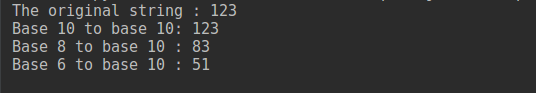## ValueError when converting String to int

While converting from string to int you may get `ValueError` exception. This exception occurs if the string you want to convert does not represent any numbers. Suppose, you want to convert a hexadecimal number to an integer. But you did not pass argument base=16 in the int() function. It will raise a `ValueError` exception if there is any digit that does not belong to the decimal number system. The following example will illustrate this exception while converting a string to int.

``````"""
Scenario 1: The interpreter will not raise any exception but you get wrong data
"""
num = '12'  # this is a hexadecimal value

# the variable is considered as decimal value during conversion
print('The value is :', int(num))

# the variable is considered as hexadecimal value during conversion
print('Actual value is :', int(num, base=16))

"""
Scenario 2: The interpreter will raise ValueError exception
"""

num = '1e'  # this is a hexadecimal value

# the variable is considered as hexadecimal value during conversion
print('Actual value of \'1e\' is :', int(num, base=16))

# the variable is considered as decimal value during conversion
print('The value is :', int(num))  # this will raise exception
``````

The output of the above code will be:

``````The value is : 12
Actual value is : 18
Actual value of '1e' is : 30
Traceback (most recent call last):
File "/home/imtiaz/Desktop/str2int_exception.py", line 22, in
print('The value is :', int(num))  # this will raise exception
ValueError: invalid literal for int() with base 10: '1e'
``````

## Python int to String

Converting an int to string requires no effort or checking. You just use `str()` function to do the conversion. See the following example.

``````hexadecimalValue = 0x1eff

``````

The output of the following code will be:

``````Type of hexadecimalValue : <class 'int'>
Type of hexadecimalValue now : <class 'str'>
``````

That’s all about Python convert String to int and int to string conversion. Reference: Python Official Doc

]]>
Pankaj 2022-08-03T20:14:22.933Z
<![CDATA[Python String Substring]]> tag:www.digitalocean.com,2005:/community/tutorials/python-string-substring 2022-08-03T20:14:22.596Z A substring is the part of a string. Python string provides various methods to create a substring, check if it contains a substring, index of substring etc. In this tutorial, we will look into various operations related to substrings.

## Python String Substring

Let’s first look at two different ways to create a substring.

### Create a Substring

We can create a substring using string slicing. We can use split() function to create a list of substrings based on specified delimiter.

``````s = 'My Name is Pankaj'

# create substring using slice
name = s[11:]
print(name)

# list of substrings using split
l1 = s.split()
print(l1)
``````

Output:

``````Pankaj
['My', 'Name', 'is', 'Pankaj']
``````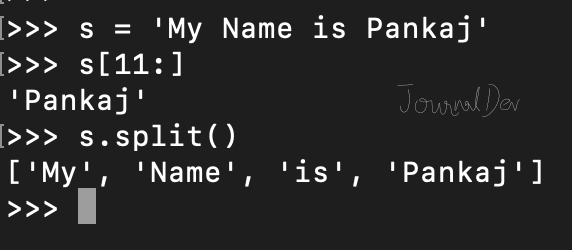### Checking if substring is found

We can use in operator or find() function to check if substring is present in the string or not.

``````s = 'My Name is Pankaj'

if 'Name' in s:
print('Substring found')

if s.find('Name') != -1:
print('Substring found')
``````

Note that find() function returns the index position of the substring if it’s found, otherwise it returns -1.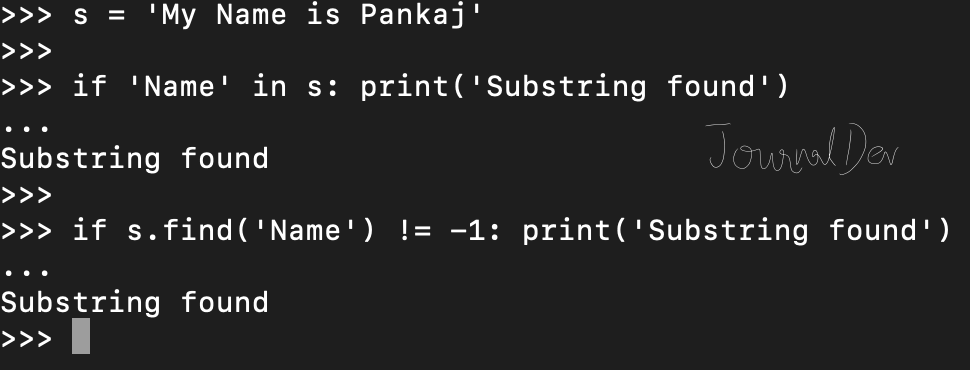### Count of Substring Occurrence

We can use count() function to find the number of occurrences of a substring in the string.

``````s = 'My Name is Pankaj'

print('Substring count =', s.count('a'))

s = 'This Is The Best Theorem'
print('Substring count =', s.count('Th'))
``````

Output:

``````Substring count = 3
Substring count = 3
``````### Find all indexes of substring

There is no built-in function to get the list of all the indexes for the substring. However, we can easily define one using find() function.

``````def find_all_indexes(input_str, substring):
l2 = []
length = len(input_str)
index = 0
while index < length:
i = input_str.find(substring, index)
if i == -1:
return l2
l2.append(i)
index = i + 1
return l2

s = 'This Is The Best Theorem'
print(find_all_indexes(s, 'Th'))
``````

Output: `[0, 8, 17]`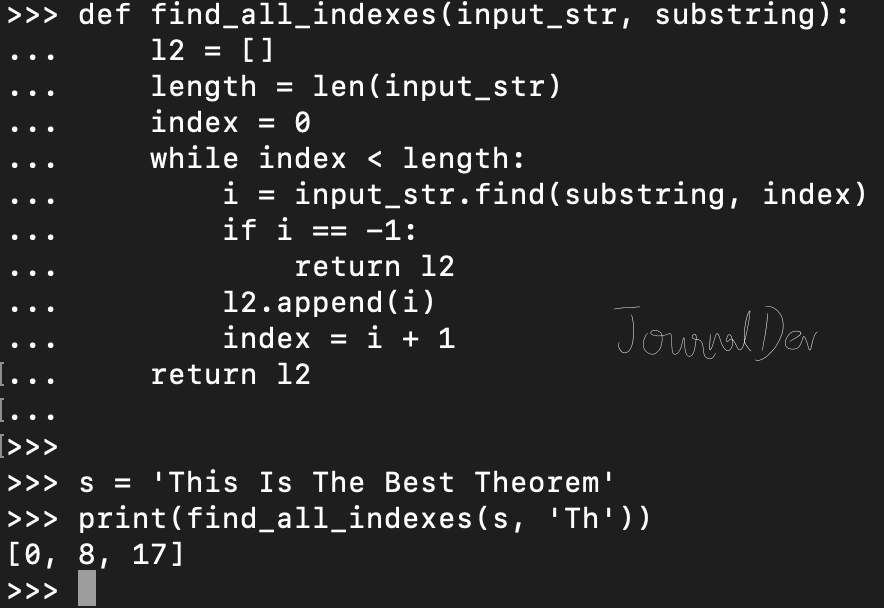You can checkout complete python script and more Python examples from our GitHub Repository.

]]>
Pankaj 2022-08-03T20:14:22.596Z
<![CDATA[Python String replace()]]> tag:www.digitalocean.com,2005:/community/tutorials/python-string-replace 2022-08-03T20:14:22.547Z Python string replace() function is used to create a string by replacing some parts of another string.

## Python String replace

Python String replace() function syntax is:

``````str.replace(old, new[, count])
``````

The original string remains unmodified. The new string is a copy of the original string with all occurrences of substring old replaced by new. If optional argument `count` is provided, then only first count occurrences are replaced. We can use this function to replace characters in a string too.

## Python String replace() example

Let’s look at some simple examples of using string replace() function.

``````s = 'Java is Nice'

# simple string replace example
str_new = s.replace('Java', 'Python')
print(str_new)

# replace character in string
s = 'dododo'
str_new = s.replace('d', 'c')
print(str_new)
``````

Output:

``````Python is Nice
cococo
``````

### Python string replace with count

``````s = 'dododo'
str_new = s.replace('d', 'c', 2)
print(str_new)
``````

Output: `cocodo`

### String replace() example with user input

``````input_str = input('Please provide input data\n')
delimiter = input('Please provide current delimiter\n')
delimiter_new = input('Please provide new delimiter\n')
output_str = input_str.replace(delimiter, delimiter_new)
print('Updated Data =', output_str)
``````

Output:

``````Please provide input data
a,e,i,o,u
,
:
Updated Data = a:e:i:o:u
``````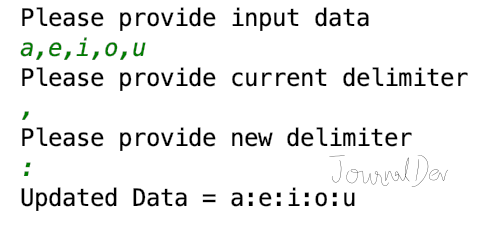We can also use str.replace() function as shown below.

``````print(str.replace('abca', 'a', 'A'))
``````

Output: `AbcA`

You can checkout complete script and more Python String examples from our GitHub Repository.

Reference: API Doc

]]>
Pankaj 2022-08-03T20:14:22.547Z
<![CDATA[Python String isalnum()]]> tag:www.digitalocean.com,2005:/community/tutorials/python-string-isalnum 2022-08-03T19:33:49.954Z Python string isalnum() function returns `True` if it’s made of alphanumeric characters only. A character is alphanumeric if it’s either an alpha or a number. If the string is empty, then isalnum() returns `False`.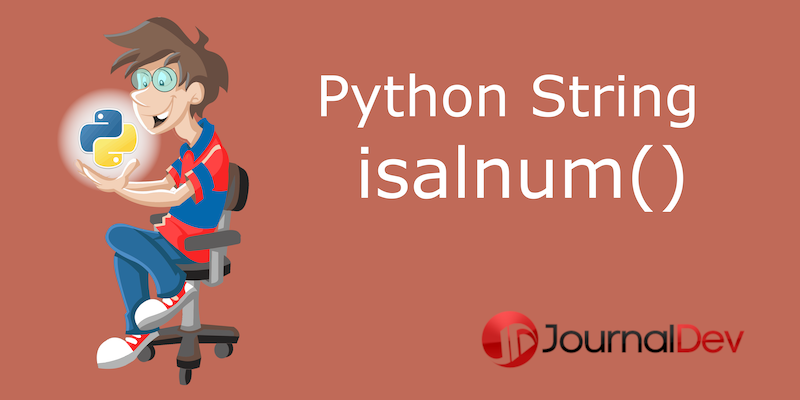## Python string isalnum() example

``````s = 'HelloWorld2019'
print(s.isalnum())
``````

Output: `True`

``````s = 'Hello World 2019'

print(s.isalnum())
``````

Output: `False` because whitespace is not an alphanumeric character.

``````s = ''
print(s.isalnum())
``````

Output: `False` because it’s an empty string.

``````s='A.B'
print(s.isalnum())

s = '10.50'
print(s.isalnum())
``````

Output:

``````False
False
``````

The string contains period (.) which is not an alphanumeric character.

``````s = 'çåøÉ'
print(s.isalnum())
``````

Output: `True` because all these are Alpha characters. Alphabetic characters are those characters defined in the Unicode character database as “Letter”, i.e., those with general category property being one of “Lm”, “Lt”, “Lu”, “Ll”, or “Lo”.

## Printing all Alphanumeric characters in Python

We can use `unicode` module to check if a character is alphanumeric or not. Here is the program to print all the alphanumeric unicode characters.

``````import unicodedata

count = 0
for codepoint in range(2 ** 16):
ch = chr(codepoint)
if ch.isalnum():
print(u'{:04x}: {} ({})'.format(codepoint, ch, unicodedata.name(ch, 'UNNAMED')))
count = count + 1
print(f'Total Number of Alphanumeric Unicode Characters = {count}')
``````

Output:

``````...
ffd7: ￗ (HALFWIDTH HANGUL LETTER YU)
ffda: ￚ (HALFWIDTH HANGUL LETTER EU)
ffdb: ￛ (HALFWIDTH HANGUL LETTER YI)
ffdc: ￜ (HALFWIDTH HANGUL LETTER I)
Total Number of Alphanumeric Unicode Characters = 49567
``````

I have provided only partial output because the number of alphanumeric unicode characters is huge.

You can checkout more Python examples from our GitHub Repository.

Reference: Official Documentation

]]>
Pankaj 2022-08-03T19:33:49.954Z
<![CDATA[Python String contains]]> tag:www.digitalocean.com,2005:/community/tutorials/python-string-contains 2022-08-03T19:33:49.799Z Python String class has `__contains__()` function that we can use to check if it contains another string or not.

## Python String contains

Python string `__contains__()` is an instance method and returns boolean value True or False depending on whether the string object contains the specified string object or not. Note that the Python string contains() method is case sensitive. Let’s look at a simple example for string __contains__() method.

``````s = 'abc'

print('s contains a =', s.__contains__('a'))
print('s contains A =', s.__contains__('A'))
print('s contains X =', s.__contains__('X'))
``````

Output:

``````s contains a = True
s contains A = False
s contains X = False
``````

We can use __contains__() function as str class method too.

``````print(str.__contains__('ABC', 'A'))
print(str.__contains__('ABC', 'D'))
``````

Output:

``````True
False
``````

Let’s look at another example where we will ask the user to enter both the strings and check if the first string contains the second string or not.

``````input_str1 = input('Please enter first input string\n')

input_str2 = input('Please enter second input string\n')

print('First Input String Contains Second String? ', input_str1.__contains__(input_str2))
``````

Output: Please enter first input string JournalDev is Nice Please enter second input string Dev First Input String Contains Second String? True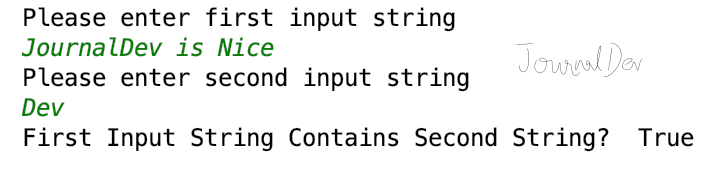You can checkout more Python string examples from our GitHub Repository.

]]>
Pankaj 2022-08-03T19:33:49.799Z
<![CDATA[Python String Append]]> tag:www.digitalocean.com,2005:/community/tutorials/python-string-append 2022-08-03T19:33:49.666Z Python string object is immutable. So every time we use + operator to concatenate two strings, a new string is created. If we have to append many strings, using + operator will unnecessarily create many temporary strings before we have the final result.

## Python String Append

Let’s look at a function to concatenate a string ‘n’ times.

``````def str_append(s, n):
output = ''
i = 0
while i < n:
output += s
i = i + 1
return output
``````

Note that I am defining this function to showcase the usage of + operator. I will later use timeit module to test the performance. If you simply want to concatenate a string ‘n’ times, you can do it easily using `s = 'Hi' * 10`.

Another way to perform string append operation is by creating a list and appending strings to the list. Then use string join() function to merge them together to get the result string.

``````def str_append_list_join(s, n):
l1 = []
i = 0
while i < n:
l1.append(s)
i += 1
return ''.join(l1)
``````

Let’s test these methods to make sure they are working as expected.

``````if __name__ == "__main__":
print('Append using + operator:', str_append('Hi', 10))
print('Append using list and join():', str_append_list_join('Hi', 10))
# use below for this case, above methods are created so that we can
# check performance using timeit module
print('Append using * operator:', 'Hi' * 10)
``````

Output:

``````Append using + operator: HiHiHiHiHiHiHiHiHiHi
Append using list and join(): HiHiHiHiHiHiHiHiHiHi
Append using * operator: HiHiHiHiHiHiHiHiHiHi
``````

## Best way to append strings in Python

I have both the methods defined in `string_append.py` file. Let’s use timeit module to check their performance.

``````\$ python3.7 -m timeit --number 1000 --unit usec 'import string_append' 'string_append.str_append("Hello", 1000)'
1000 loops, best of 5: 174 usec per loop
\$ python3.7 -m timeit --number 1000 --unit usec 'import string_append' 'string_append.str_append_list_join("Hello", 1000)'
1000 loops, best of 5: 140 usec per loop

\$ python3.7 -m timeit --number 1000 --unit usec 'import string_append' 'string_append.str_append("Hi", 1000)'
1000 loops, best of 5: 165 usec per loop
\$ python3.7 -m timeit --number 1000 --unit usec 'import string_append' 'string_append.str_append_list_join("Hi", 1000)'
1000 loops, best of 5: 139 usec per loop
``````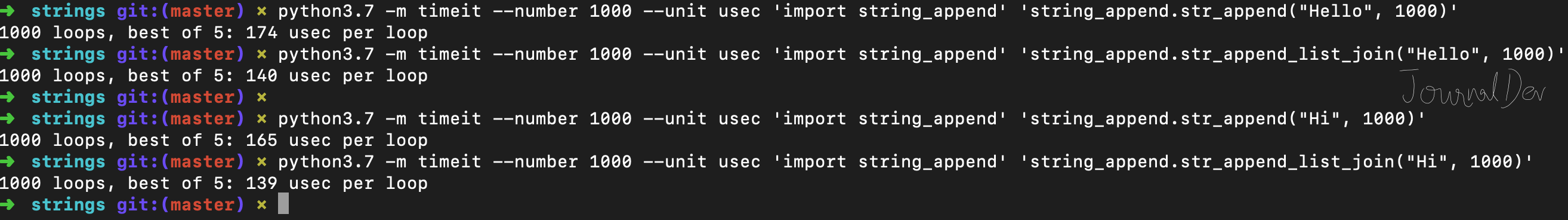## Summary

If there are few strings, then you can use any method to append them. From a readability perspective, using + operator seems better for few strings. However, if you have to append a lot of string, then you should use the list and join() function.

You can checkout complete python script and more Python examples from our GitHub Repository.

]]>
Pankaj 2022-08-03T19:33:49.666Z
<![CDATA[Python f-strings - PEP 498 - Literal String Interpolation]]> tag:www.digitalocean.com,2005:/community/tutorials/python-f-strings-literal-string-interpolation 2022-08-03T19:33:45.697Z Python f-strings or formatted strings are the new way to format strings. This feature was introduced in Python 3.6 under PEP-498. It’s also called literal string interpolation.

## Why do we need f-strings?

Python provides various ways to format a string. Let’s quickly look at them and what are the issues they have.

• % formatting - great for simple formatting but limited support for strings, ints, doubles only. We can’t use it with objects.

• Template Strings - it’s very basic. Template strings work with keyword arguments like dictionary only. We are not allowed to call any function and arguments must be strings.

• String format() - Python String format() function was introduced to overcome the issues and limited features of %-formatting and template strings. However, it’s too verbose. Let’s look at its verbosity with a simple example.

``````>>> age = 4 * 10
>>> 'My age is {age}.'.format(age=age)
'My age is 40.'
``````

Python f-strings works almost similar like format() function but removes all the verbosity that format() function has. Let’s see how easily we can format the above string using f-strings.

``````>>> f'My age is {age}'
'My age is 40.'
``````

Python f-strings is introduced to have minimal syntax for string formatting. The expressions are evaluated at runtime. If you are using Python 3.6 or higher version, you should use f-strings for all your string formatting requirements.

## Python f-strings examples

Let’s look at a simple example of f-strings.

``````name = 'Pankaj'
age = 34

f_string = f'My Name is {name} and my age is {age}'

print(f_string)
print(F'My Name is {name} and my age is {age}')  # f and F are same

name = 'David'
age = 40

# f_string is already evaluated and won't change now
print(f_string)
``````

Output:

``````My Name is Pankaj and my age is 34
My Name is Pankaj and my age is 34
My Name is Pankaj and my age is 34
``````

Python executes statements one by one and once f-string expressions are evaluated, they don’t change even if the expression value changes. That’s why in the above code snippets, f_string value remains same even after ‘name’ and ‘age’ variable has changed in the latter part of the program.

### 1. f-strings with expressions and conversions

We can use f-strings to convert datetime to a specific format. We can also run mathematical expressions in f-strings.

``````from datetime import datetime

name = 'David'
age = 40
d = datetime.now()

print(f'Age after five years will be {age+5}')  # age = 40
print(f'Name with quotes = {name!r}')  # name = David
print(f'Default Formatted Date = {d}')
print(f'Custom Formatted Date = {d:%m/%d/%Y}')
``````

Output:

``````Age after five years will be 45
Name with quotes = 'David'
Default Formatted Date = 2018-10-10 11:47:12.818831
Custom Formatted Date = 10/10/2018
``````

### 2. f-strings support raw strings

We can create raw strings using f-strings too.

``````print(f'Default Formatted Date:\n{d}')
print(fr'Default Formatted Date:\n {d}')
``````

Output:

``````Default Formatted Date:
2018-10-10 11:47:12.818831
Default Formatted Date:\n 2018-10-10 11:47:12.818831
``````

### 3. f-strings with objects and attributes

We can access object attributes too in f-strings.

``````class Employee:
id = 0
name = ''

def __init__(self, i, n):
self.id = i
self.name = n

def __str__(self):
return f'E[id={self.id}, name={self.name}]'

emp = Employee(10, 'Pankaj')
print(emp)

print(f'Employee: {emp}\nName is {emp.name} and id is {emp.id}')
``````

Output:

``````E[id=10, name=Pankaj]
Employee: E[id=10, name=Pankaj]
Name is Pankaj and id is 10
``````

### 4. f-strings calling functions

We can call functions in f-strings formatting too.

``````def add(x, y):
return x + y

``````

Output: `Sum(10,20) = 30`

### 5. f-string with whitespaces

If there are leading or trailing whitespaces in the expression, they are ignored. If the literal string part contains whitespaces then they are preserved.

``````>>> age = 4 * 20
>>> f'   Age = {  age   }  '
'   Age = 80  '
``````

### 6. Lambda expressions with f-strings

We can use lambda expressions insidef-string expressions too.

``````x = -20.45
print(f'Lambda Example: {(lambda x: abs(x)) (x)}')

print(f'Lambda Square Example: {(lambda x: pow(x, 2)) (5)}')
``````

Output:

``````Lambda Example: 20.45
Lambda Square Example: 25
``````

### 7. f-strings miscellaneous examples

Let’s look at some miscellaneous examples of Python f-strings.

``````print(f'{"quoted string"}')
print(f'{{ {4*10} }}')
print(f'{{{4*10}}}')
``````

Output:

``````quoted string
{ 40 }
{40}
``````

That’s all for python formatted strings aka f-strings.

You can checkout complete python script and more Python examples from our GitHub Repository.

Reference: PEP-498, Official Documentation

]]>
Pankaj 2022-08-03T19:33:45.697Z
<![CDATA[Python String equals]]> tag:www.digitalocean.com,2005:/community/tutorials/python-string-equals 2022-08-03T18:46:47.561Z Python strings equality can be checked using `==` operator or `__eq__()` function. Python strings are case sensitive, so these equality check methods are also case sensitive.

## Python String equals

Let’s look at some examples to check if two strings are equal or not.

``````s1 = 'Apple'

s2 = 'Apple'

s3 = 'apple'

# case sensitive equals check
if s1 == s2:
print('s1 and s2 are equal.')

if s1.__eq__(s2):
print('s1 and s2 are equal.')
``````

Output:

``````s1 and s2 are equal.
s1 and s2 are equal.
``````

If you want to perform inequality check, you can use `!=` operator.

``````if s1 != s3:
print('s1 and s3 are not equal')
``````

Output: `s1 and s3 are not equal`

## Python String equals case-insensitive check

Sometimes we don’t care about the case while checking if two strings are equal, we can use `casefold()`, `lower()` or `upper()` functions for case-insensitive equality check.

``````if s1.casefold() == s3.casefold():
print(s1.casefold())
print(s3.casefold())
print('s1 and s3 are equal in case-insensitive comparison')

if s1.lower() == s3.lower():
print(s1.lower())
print(s3.lower())
print('s1 and s3 are equal in case-insensitive comparison')

if s1.upper() == s3.upper():
print(s1.upper())
print(s3.upper())
print('s1 and s3 are equal in case-insensitive comparison')
``````

Output:

``````apple
apple
s1 and s3 are equal in case-insensitive comparison
apple
apple
s1 and s3 are equal in case-insensitive comparison
APPLE
APPLE
s1 and s3 are equal in case-insensitive comparison
``````

## Python String equals with special characters

Let’s look at some examples where strings contain special characters.

``````s1 = '\$#ç∂'
s2 = '\$#ç∂'

print('s1 == s2?', s1 == s2)
print('s1 != s2?', s1 != s2)
print('s1.lower() == s2.lower()?', s1.lower() == s2.lower())
print('s1.upper() == s2.upper()?', s1.upper() == s2.upper())
print('s1.casefold() == s2.casefold()?', s1.casefold() == s2.casefold())
``````

Output:

``````s1 == s2? True
s1 != s2? False
s1.lower() == s2.lower()? True
s1.upper() == s2.upper()? True
s1.casefold() == s2.casefold()? True
``````

That’s all for checking if two strings are equal or not in Python.

You can checkout complete script and more Python String examples from our GitHub Repository.

]]>
Pankaj 2022-08-03T18:46:47.561Z
<![CDATA[Python String Concatenation]]> tag:www.digitalocean.com,2005:/community/tutorials/python-string-concatenation 2022-08-03T18:46:47.501Z String Concatenation is a very common operation in programming. Python String Concatenation can be done using various ways. This tutorial is aimed to explore different ways to concatenate strings in a python program.

## Python String Concatenation

We can perform string concatenation using following ways:

• Using + operator
• Using join() method
• Using % operator
• Using format() function
• Using f-string (Literal String Interpolation)

### String Concatenation using + Operator

This is the most simple way of string concatenation. Let’s look at a simple example.

``````s1 = 'Apple'
s2 = 'Pie'
s3 = 'Sauce'

s4 = s1 + s2 + s3

print(s4)
``````

Output: `ApplePieSauce` Let’s look at another example where we will get two strings from user input and concatenate them.

``````s1 = input('Please enter the first string:\n')
s2 = input('Please enter the second string:\n')

print('Concatenated String =', s1 + s2)
``````

Output:

``````Please enter the first string:
Hello
World
Concatenated String = HelloWorld
``````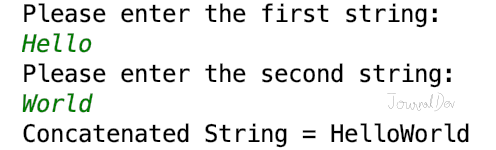It’s very easy to use + operator for string concatenation. However, the arguments must be a string.

``````>>>'Hello' + 4
Traceback (most recent call last):
File "<input>", line 1, in
TypeError: can only concatenate str (not "int") to str
``````

We can use str() function to get the string representation of an object. Let’s see how to concatenate a string to integer or another object.

``````print('Hello' + str(4))

class Data:
id = 0

def __init__(self, i):
self.id = i

def __str__(self):
return 'Data[' + str(self.id) + ']'

print('Hello ' + str(Data(10)))
``````

Output:

``````Hello4
Hello Data
``````

The biggest issue with + operator is that we can’t add any separator or delimiter between strings. For example, if we have to concatenate “Hello” and “World” with a whitespace separator, we will have to write it as `"Hello" + " " + "World"`.

### String concatenation using join() function

We can use join() function to concatenate string with a separator. It’s useful when we have a sequence of strings, for example list or tuple of strings. If you don’t want a separator, then use join() function with an empty string.

``````s1 = 'Hello'
s2 = 'World'

print('Concatenated String using join() =', "".join([s1, s2]))

print('Concatenated String using join() and whitespaces =', " ".join([s1, s2]))
``````

Output:

``````Concatenated String using join() = HelloWorld
Concatenated String using join() and spaces = Hello World
``````

### String Concatenation using % Operator

We can use % operator for string formatting, it can be used for string concatenation too. It’s useful when we want to concatenate strings and perform simple formatting.

``````s1 = 'Hello'
s2 = 'World'

s3 = "%s %s" % (s1, s2)
print('String Concatenation using % Operator =', s3)

s3 = "%s %s from JournalDev - %d" % (s1, s2, 2018)
print('String Concatenation using % Operator with Formatting =', s3)
``````

Output:

``````String Concatenation using % Operator = Hello World
String Concatenation using % Operator with Formatting = Hello World from JournalDev - 2018
``````

### String Concatenation using format() function

We can use string format() function for string concatenation and formatting too.

``````s1 = 'Hello'
s2 = 'World'

s3 = "{}-{}".format(s1, s2)
print('String Concatenation using format() =', s3)

s3 = "{in1} {in2}".format(in1=s1, in2=s2)
print('String Concatenation using format() =', s3)
``````

Output:

``````String Concatenation using format() = Hello-World
String Concatenation using format() = Hello World
``````

Python String format() function is very powerful, using it just for concatenation of strings is not its proper use.

### String Concatenation using f-string

If you are using Python 3.6+, you can use f-string for string concatenation too. It’s a new way to format strings and introduced in PEP 498 - Literal String Interpolation.

``````s1 = 'Hello'
s2 = 'World'

s3 = f'{s1} {s2}'
print('String Concatenation using f-string =', s3)

name = 'Pankaj'
age = 34
d = Data(10)

print(f'{name} age is {age} and d={d}')
``````

Output:

``````String Concatenation using f-string = Hello World
Pankaj age is 34 and d=Data
``````

Python f-string is cleaner and easier to write when compared to format() function. It also calls str() function when an object argument is used as field replacement.

## Conclusion

Python String formatting can be done in several ways. Use them based on your requirements. If you have to concatenate sequence of strings with a delimited, then use join() function. If some formatting is also required with concatenation, then use format() function or f-string. Note that f-string can be used with Python 3.6 or above versions.

You can checkout complete python script and more Python examples from our GitHub Repository.

]]>
Pankaj 2022-08-03T18:46:47.501Z
<![CDATA[Python String Module]]> tag:www.digitalocean.com,2005:/community/tutorials/python-string-module 2022-08-03T18:17:41.656Z Python String module contains some constants, utility function, and classes for string manipulation.

## Python String Module

It’s a built-in module and we have to import it before using any of its constants and classes.

### String Module Constants

Let’s look at the constants defined in the string module.

``````import string

# string module constants
print(string.ascii_letters)
print(string.ascii_lowercase)
print(string.ascii_uppercase)
print(string.digits)
print(string.hexdigits)
print(string.whitespace)  # ' \t\n\r\x0b\x0c'
print(string.punctuation)
``````

Output:

``````abcdefghijklmnopqrstuvwxyzABCDEFGHIJKLMNOPQRSTUVWXYZ
abcdefghijklmnopqrstuvwxyz
ABCDEFGHIJKLMNOPQRSTUVWXYZ
0123456789
0123456789abcdefABCDEF

!"#\$%&'()*+,-./:;?@[\]^_`{|}~
``````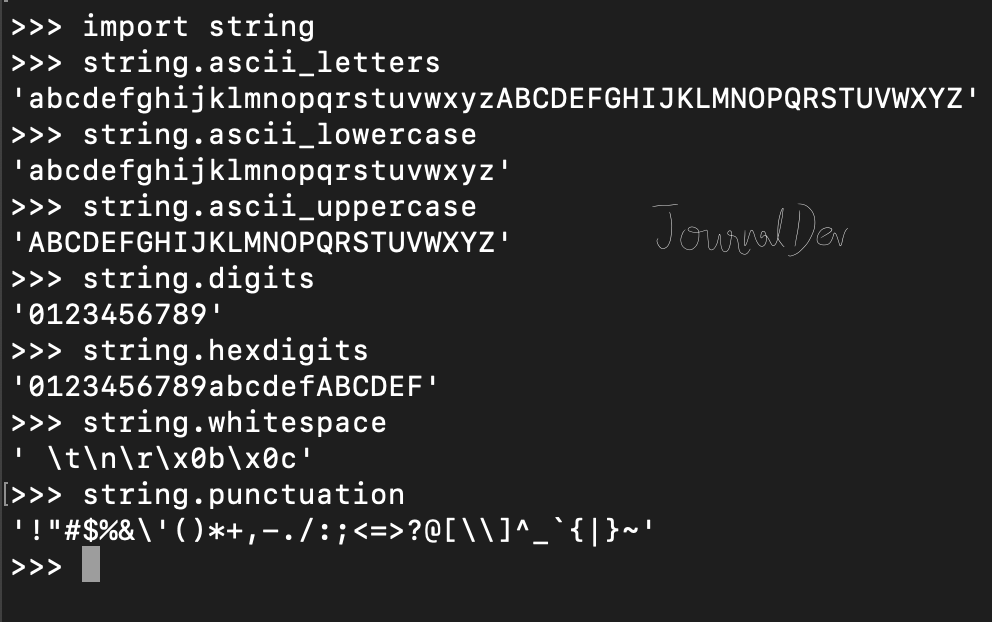### string capwords() function

Python string module contains a single utility function - capwords(s, sep=None). This function split the specified string into words using str.split(). Then it capitalizes each word using `str.capitalize()` function. Finally, it joins the capitalized words using str.join(). If the optional argument sep is not provided or None, then leading and trailing whitespaces are removed and words are separated with single whitespace. If it’s provided then the separator is used to split and join the words.

``````s = '  Welcome TO  \n\n JournalDev '
print(string.capwords(s))
``````

Output: `Welcome To Journaldev`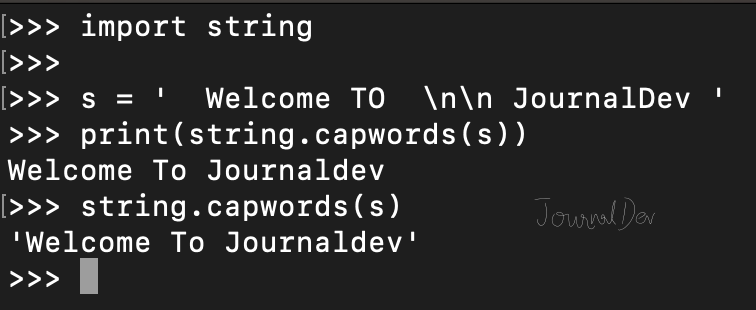## Python String Module Classes

Python string module contains two classes - Formatter and Template.

### Formatter

It behaves exactly same as str.format() function. This class become useful if you want to subclass it and define your own format string syntax. Let’s look at a simple example of using Formatter class.

``````from string import Formatter

formatter = Formatter()
print(formatter.format('{website}', website='JournalDev'))
print(formatter.format('{} {website}', 'Welcome to', website='JournalDev'))

# format() behaves in similar manner
print('{} {website}'.format('Welcome to', website='JournalDev'))
``````

Output:

``````Welcome to JournalDev
Welcome to JournalDev
``````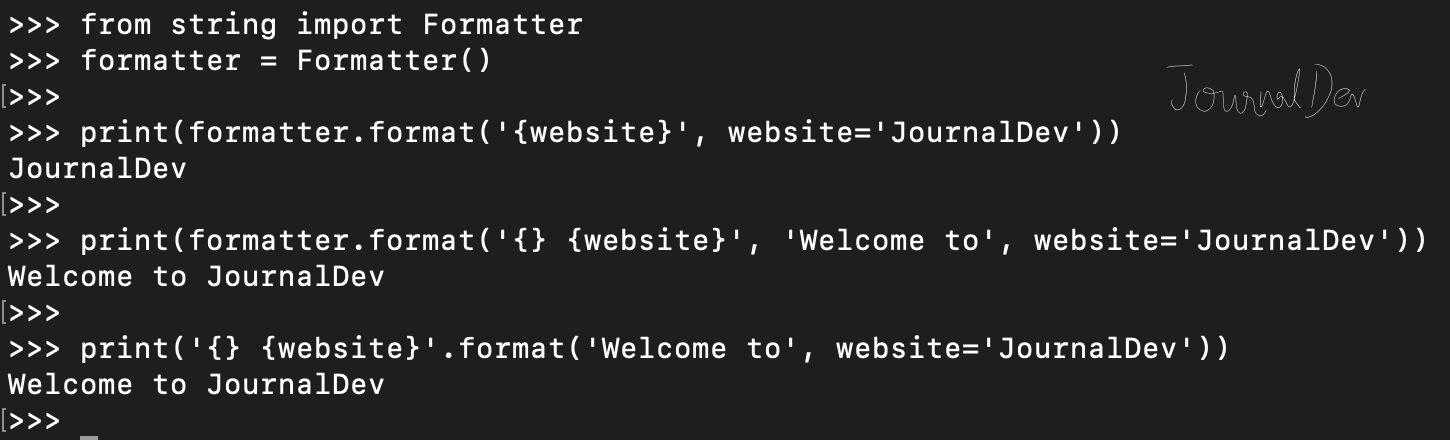### Template

This class is used to create a string template for simpler string substitutions as described in PEP 292. It’s useful in implementing internationalization (i18n) in an application where we don’t need complex formatting rules.

``````from string import Template

t = Template('\$name is the \$title of \$company')
s = t.substitute(name='Pankaj', title='Founder', company='JournalDev.')
print(s)
``````

Output: `Pankaj is the Founder of JournalDev.`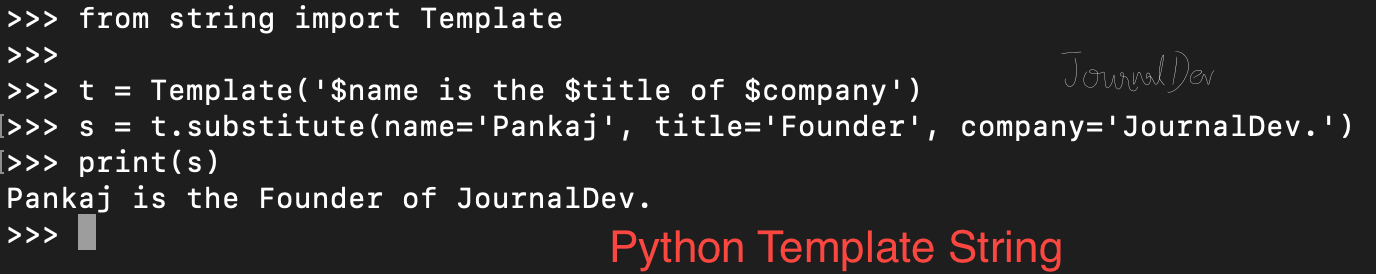You can checkout complete python script and more Python examples from our GitHub Repository.

Reference: Official Documentation

]]>
Pankaj 2022-08-03T18:17:41.656Z
<![CDATA[Python String Functions]]> tag:www.digitalocean.com,2005:/community/tutorials/python-string-functions 2022-08-03T18:17:41.572Z Python provides a lot of built-in functions to manipulate strings. Python String is immutable, so all these functions return a new string and the original string remains unchanged.

## Python String Functions

There are many functions to operate on String. However, it’s not feasible to remember all of them. So here I am dividing them into different categories.

• Must Know String Functions
• Good to Know String Functions
• Miscellaneous String Functions
• Built-in Functions that work on String
• Useful String Operations

## Must Know String Functions

Function Description
format() It’s used to create a formatted string from the template string and the supplied values.
split() Python string split() function is used to split a string into the list of strings based on a delimiter.
join() This function returns a new string that is the concatenation of the strings in iterable with string object as a delimiter.
strip() Used to trim whitespaces from the string object.
format_map() Python string format_map() function returns a formatted version of the string using substitutions from the mapping provided.
upper() We can convert a string to uppercase in Python using str.upper() function.
lower() This function creates a new string in lowercase.
replace() Python string replace() function is used to create a new string by replacing some parts of another string.
find() Python String find() method is used to find the index of a substring in a string.
translate() Python String translate() function returns a new string with each character in the string replaced using the given translation table.

## Good to Know String Functions

Function Description
encode() Python string encode() function is used to encode the string using the provided encoding.
count() Python String count() function returns the number of occurrences of a substring in the given string.
startswith() Python string startswith() function returns True if the string starts with the given prefix, otherwise it returns False.
endswith() Python string endswith() function returns True if the string ends with the given suffix, otherwise it returns False.
capitalize() Python String capitalize() function returns the capitalized version of the string.
center() Python string center() function returns a centered string of specified size.
casefold() Python string casefold() function returns a casefolded copy of the string. This function is used to perform case-insensitive string comparison.
expandtabs() Python string expandtabs() function returns a new string with tab characters (\t) replaced with one or more whitespaces.
index() Python String index() function returns the lowest index where the specified substring is found.
__contains__() Python String class has __contains__() function that we can use to check if it contains another string or not. We can also use “in” operator to perform this check.

## Miscellaneous String Functions

Function Description
isalnum() Python string isalnum() function returns True if it’s made of alphanumeric characters only.
isalpha() Python String isalpha() function returns True if all the characters in the string are alphabets, otherwise False.
isdecimal() Python String isdecimal() function returns True if all the characters in the string are decimal characters, otherwise False.
isdigit() Python String isdigit() function returns True if all the characters in the string are digits, otherwise False.
isidentifier() Python String isidentifier() function returns True if the string is a valid identifier according to the Python language definition.
islower() Python String islower() returns True if all cased characters in the string are lowercase and there is at least one cased character, otherwise it returns False.
isnumeric() Python String isnumeric() function returns True if all the characters in the string are numeric, otherwise False. If the string is empty, then this function returns False.
isprintable() Python String isprintable() function returns True if all characters in the string are printable or the string is empty, False otherwise.
isspace() Python String isspace() function returns True if there are only whitespace characters in the string, otherwise it returns False.
istitle() Python String istitle() returns True if the string is title cased and not empty, otherwise it returns False.
isupper() Python String isupper() function returns True if all the cased characters are in Uppercase.
rjust(), ljust() Utility functions to create a new string of specified length from the source string with right and left justification.
swapcase() Python String swapcase() function returns a new string with uppercase characters converted to lowercase and vice versa.
partition() Python String partition() function splits a string based on a separator into a tuple with three strings.
splitlines() Python String splitlines() function returns the list of lines in the string.
title() Python String title() function returns a title cased version of the string.
zfill() Python String zfill(width) function returns a new string of specified width. The string is filled with 0 on the left side to create the specified width.

## Built-in Functions that work on String

Function Description
len() Python String length can be determined by using built-in len() function.
ascii() Python ascii() function returns the string representation of the object. This function internally calls repr() function and before returning the representation string, escapes the non-ASCII characters using \x, \u or \U escapes.
bool() Python bool() function returns Boolean value for an object. The bool class has only two instances – True and False.
bytearray() Python bytearray() function returns a bytearray object that contains the array of bytes from the input source.
bytes() This function returns bytes object that is an immutable sequence of integers in the range 0 <= x < 256.
ord() Python ord() function takes string argument of a single Unicode character and return its integer Unicode code point value.
enumerate() Python enumerate function takes a sequence, and then make each element of the sequence into a tuple.
float() As the name says, python float() function returns a floating point number from the input argument.
hash() This function returns the hash value of the given object.
id() Python id() function returns the “identity” of the object. The identity of an object is an integer, which is guaranteed to be unique and constant for this object during its lifetime.
int() Python int() function returns an integer object from the specified input. The returned int object will always be in base 10.
map() Python map() function is used to apply a function on all the elements of specified iterable and return map object.
print() Python print() function is used to print data into console.
slice() Python slice() function returns a slice object representing the set of indices specified by range(start, stop, step).
type() This function returns the type of the object.

## Do I need to remember all of them?

Nobody can remember all of them. You can always find them in your IDE. Below image is from my PyCharm IDE builtins.py file.

## Have I listed All the Python String Methods?

I have listed almost all the important python string methods. However, some of them might have missed. This list is updated till Python 3.7. So any function coming up in later releases is not listed here, at least not right now. If you think that I have missed some important function, please respond in comments and I will add them too.

]]>
Pankaj 2022-08-03T18:17:41.572Z
<![CDATA[Python String encode() decode()]]> tag:www.digitalocean.com,2005:/community/tutorials/python-string-encode-decode 2022-08-03T18:17:41.496Z Python String encode()

Python string encode() function is used to encode the string using the provided encoding. This function returns the bytes object. If we don’t provide encoding, “utf-8” encoding is used as default.

## Python Bytes decode()

Python bytes decode() function is used to convert bytes to string object. Both these functions allow us to specify the error handling scheme to use for encoding/decoding errors. The default is ‘strict’ meaning that encoding errors raise a UnicodeEncodeError. Some other possible values are ‘ignore’, ‘replace’ and ‘xmlcharrefreplace’. Let’s look at a simple example of python string encode() decode() functions.

``````str_original = 'Hello'

bytes_encoded = str_original.encode(encoding='utf-8')
print(type(bytes_encoded))

str_decoded = bytes_encoded.decode()
print(type(str_decoded))

print('Encoded bytes =', bytes_encoded)
print('Decoded String =', str_decoded)
print('str_original equals str_decoded =', str_original == str_decoded)
``````

Output:

``````<class 'bytes'>
<class 'str'>
Encoded bytes = b'Hello'
Decoded String = Hello
str_original equals str_decoded = True
``````

Above example doesn’t clearly demonstrate the use of encoding. Let’s look at another example where we will get inputs from the user and then encode it. We will have some special characters in the input string entered by the user.

``````str_original = input('Please enter string data:\n')

bytes_encoded = str_original.encode()

str_decoded = bytes_encoded.decode()

print('Encoded bytes =', bytes_encoded)
print('Decoded String =', str_decoded)
print('str_original equals str_decoded =', str_original == str_decoded)
``````

Output: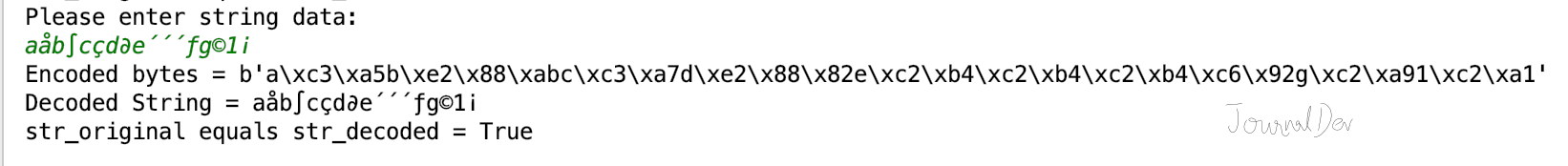``````Please enter string data:
Encoded bytes = b'a\xc3\xa5b\xe2\x88\xabc\xc3\xa7d\xe2\x88\x82e\xc2\xb4\xc2\xb4\xc2\xb4\xc6\x92g\xc2\xa91\xc2\xa1'
str_original equals str_decoded = True
``````

You can checkout complete python script and more Python examples from our GitHub Repository.

Reference: str.encode() API Doc, bytes.decode() API Doc

]]>
Pankaj 2022-08-03T18:17:41.496Z
<![CDATA[An Introduction to Working with Strings in Python 3]]> tag:www.digitalocean.com,2005:/community/tutorials/an-introduction-to-working-with-strings-in-python-3 2021-08-20T16:36:54.403Z Introduction

A string is a sequence of one or more characters (letters, numbers, symbols) that can be either a constant or a variable. Made up of Unicode, strings are immutable sequences, meaning they are unchanging.

Because text is such a common form of data that we use in everyday life, the string data type is a very important building block of programming.

This Python tutorial will go over how to create and print strings, how to concatenate and replicate strings, and how to store strings in variables.

## Prerequisites

You should have Python 3 installed and a programming environment set up on your computer or server. If you don’t have a programming environment set up, you can refer to the installation and setup guides for a local programming environment or for a programming environment on your server appropriate for your operating system (Ubuntu, CentOS, Debian, etc.)

## Creating and Printing Strings

Strings exist within either single quotes `'` or double quotes `"` in Python, so to create a string, enclose a sequence of characters in one or the other:

Info: To follow along with the example code in this tutorial, open a Python interactive shell on your local system by running the `python3` command. Then you can copy, paste, or edit the examples by adding them after the `>>>` prompt.

``````'This is a string in single quotes.'
``````
``````"This is a string in double quotes."
``````

You can choose to use either single quotes or double quotes, but whichever you decide on you should be consistent within a program.

We can print out strings by calling the `print()` function:

``````print("Let's print out this string.")
``````
``````OutputLet's print out this string.
``````

With an understanding of how strings are formatted in Python, let’s take a look at how we can work with and manipulate strings in programs.

## String Concatenation

Concatenation means joining strings together end-to-end to create a new string. To concatenate strings, we use the `+` operator. Keep in mind that when we work with numbers, `+` will be an operator for addition, but when used with strings it is a joining operator.

Let’s combine the strings `"Sammy"` and `"Shark"` together with concatenation through a `print()` statement:

``````print("Sammy" + "Shark")
``````
``````OutputSammyShark
``````

If we would like a whitespace between the two strings, we can include the whitespace within a string, like after the word “Sammy”:

``````print("Sammy " + "Shark")
``````
``````OutputSammy Shark
``````

Be sure not to use the `+` operator between two different data types. We can’t concatenate strings and integers together, for instance. So, if we try to write:

``````print("Sammy" + 27)
``````

We will receive the following error:

``````OutputTypeError: Can't convert 'int' object to str implicitly
``````

If we wanted to create the string `"Sammy27"`, we could do so by putting the number `27` in quotes (`"27"`) so that it is no longer an integer but is instead a string. Converting numbers to strings for concatenation can be useful when dealing with zip codes or phone numbers, for example, as we don’t want to perform addition between a country code and an area code, but we do want them to stay together.

When we combine two or more strings through concatenation we are creating a new string that we can use throughout our program.

##String Replication

There may be times when you need to use Python to automate tasks, and one way you may do this is through repeating a string several times. You can do so with the `*` operator. Like the `+` operator, the `*` operator has a different use when used with numbers, where it is the operator for multiplication. When used with one string and one integer, `*` is the string replication operator, repeating a single string however many times you would like through the integer you provide.

Let’s print out “Sammy” 9 times without typing out “Sammy” 9 times with the `*` operator:

``````print("Sammy" * 9)
``````
``````OutputSammySammySammySammySammySammySammySammySammy
``````

With string replication, we can repeat the single string value the amount of times equivalent to the integer value.

## Storing Strings in Variables

Variables are symbols that you can use to store data in a program. You can think of them as an empty box that you fill with some data or value. Strings are data, so we can use them to fill up a variable. Declaring strings as variables can make it easier for us to work with strings throughout our Python programs.

To store a string inside a variable, we need to assign a variable to a string. In this case let’s declare `my_str` as our variable:

``````my_str = "Sammy likes declaring strings."
``````

Now that we have the variable `my_str` set to that particular string, we can print the variable like so:

``````print(my_str)
``````

And we will receive the following output:

``````OutputSammy likes declaring strings.
``````

By using variables to stand in for strings, we do not have to retype a string each time we want to use it, making it better for us to work with and manipulate strings within our programs.

## Conclusion

This tutorial went over the basics of working with the string data type in the Python 3 programming language. Creating and printing strings, concatenating and replicating strings, and storing strings in variables will provide you with the fundamentals to use strings in your Python 3 programs.

Continue learning more about strings by taking a look at the following tutorials:

]]>
Lisa Tagliaferri 2021-08-20T16:36:54.403Z